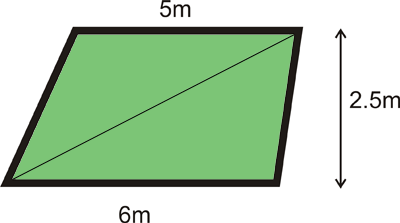SEARCH HOMEMath Central Quandaries & QueriesQuestion from maureen, a teacher: the question asks for the area of a trapezoid with parallel sides 5m and 6m and height of 2.5m I have done it by dividing the trapezoid into 2 triangles and reach the same answer as my manual---11.25 sq. m My question...how would you illustrate this? I read this to mean to sets of parallel sides, which would mean it is a parallelogram and not a trapezoid. Any suggestions?Well, you did get the right answer (see sketch) but there is only one pair of parallel sides - one of them 5m long, one 6m long. Your interpretation is more or less plausible, but it would not lead to a soluble problem, as there would be no way of telling which pair of edges the height was measured perpendicular to.Good Hunting!
RDMath Central is supported by the University of Regina and The Pacific Institute for the Mathematical Sciences.